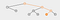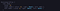# Inserting New Node To Binary Search Tree

I will talk about inserting a Binary Tree in this article. We will see all the process of inserting in a value on our Binary Tree. In the beginning we have to create a new node, then we should find the correct spot for that new node depending on the value of the number. If you check the diagram you can realize how we can find the right place for our new node in our binary tree.

Let’s say we are planning to add number(15) in our Binary Tree. How would we check number(15) and how do we compare number(14) ?

Since the number which we are trying to insert into the Binary Tree is greater than the number(14) has to place somewhere on the right side of the number(14). If you remember we already explain how to set our Binary tree in my previous article.

When we go to one level down on the right side of the node and compare our new node(15) with number(16). Obviously Number(15) smaller than number(16). That means if there is no child node on the left side of number(16), our new node will be placed there.

We can also get advantage of VisualGo Diagram to see new nodes placing steps below. Let’s say we want to insert number(84) and see which direction is available for our new node to be placed.VisualGo Diagram

I think we are pretty clear about how to find a place for our new node. Based on the examples above, I tried to explain each step to find the right place to store our new node. But we have not done it with code yet. Since we consider ourselves a Software engineer we should be very comfortable when we creating our data structure as a Binary tree.

INSERTING NEW NODE TO TREE

Next step is inserting a new node to our Binary tree with coding. Here is the pseudo code for inserting new nodes in our binary tree. We basically follow these steps when we insert in our diagram. We should take the same steps like we did for diagrams but we will do it with coding this time.

Pseudo Inserting A Node

Steps - Iteratively or Recursively

• Create a new node

Check to see if there is a node to the right

If there is, move to that node and repeat these steps

If there is not, add that node as the right property

• If it is less
Check to see if there is a node to the left
If there is, move to that node and repeat these steps
If there is not, add that nodes as the left property
`class Node {constructor(value) {    this.value = value;    this.right = null;    this.left = null;  }}class BinarySearchTree {    constructor() {        this.root = null; }insert(value) {     var newNode = new Node(value);    if (this.root === null) {        this.root = newNode;        return this;    } else {      var current = this.root      while (true) {      if (value < current.value) {            if (current.left === null) {               current.left = newNode;                return this    } else {        current = current.left;    }   } else if (value > current.value) {       if (current.right === null) {          current.right = newNode;          return this   } else {       current = current.right       }          }        }    }   }}var tree = new BinarySearchTree();tree.insert(10)tree.insert(5)tree.insert(13)tree.insert(11)tree.insert(2)tree.insert(16)tree.insert(7)console.log(tree)`Console

Let’s Break Down The Code

As you see in the first part we have a node class which I mentioned on my previous blog about trees. We have also value Left and Right set as null.

We have another Binary Search Tree Class(BTS) with root set as null.

It’s very hard to visualize how this will look in our tree. It’s not easy like Linked lists. You insert something that should go afterwards and end up with a linear list with a tree. It would be very hard to keep track of where the new nodes should go.

After we initialized our class as above we go and write an insert method which takes value. We are creating a New Node and passing the value. After that we should check if there is a root already. If there is not a root we will set our new Node as root in the beginning. Then we return a new Node.

else

We are checking if the value we are trying to insert is less than or greater than number (14) If it is less than (14 )we will go and check if there is any place available to put our new node on the left. Otherwise we will do the same thing for right side as well. If there is another node on the left or right side then we will compare one more time if the new node is less than or greater than the node on the tree. Basically we are looping and finding available places for our new node to store. As you see I also created a variable current and current would be our starting points and set to root. You can think about it like we are setting our starting point in a linked list as a head on Trees. We are setting up that as a root. And we will update our current as we go! I also set up another while loop with true here. While is going to break out of this loop using a return statement if we insert the node. While will check if the value less than or greater than root . If its value is less than current(root) it will go somewhere on the left. But we need another condition to check if there is any other node on the left side. If there is not we found our spot based on the number that we are trying to insert. We also set our Loops for statements for the right side of the tree to find the right spot for our new node.

Software Engineer | Ruby on Rails | JavaScript | HTML5 | CSS | PostgreSQL | React | Redux

## More from Soner Mezgitci

Software Engineer | Ruby on Rails | JavaScript | HTML5 | CSS | PostgreSQL | React | Redux